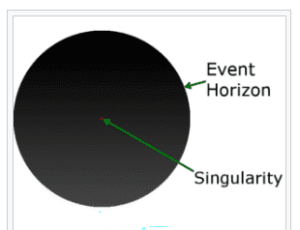Home » singularity

# singularity

« Back to Glossary Index

Singularity” has a physics definition and a mathematical definition. Both definitions are given here. They fit together nicely.

## Physics Definition of “Singularity”

A singularity in physics is a point that has an infinite value. As an infinite quantity cannot occur in our understanding of Nature, singularities are not considered real by scientists. Instead, when theories predict a singularity, scientists take it to mean that the theory has been extended beyond its applicability. A new scientific theory is needed to describe the behavior of the physical universe in this area.

There are two points in our universe that scientists call a “singularity:” One is connected with the (1) Center of a Black Hole and the other with the (2) Source of the Big Bang.

## Black Hole Singularities

General Relativity predicts that Black Holes have the mass of several stars concentrated at a point that has zero spatial volume. Mathematically, this means that at their center, Black Holes are calculated to have infinite density. This point of zero volume and infinite density is called a “singularity.”The center of a Black Hole is calculated as a singularity. [Image source: by SubstituteR – Own work, CC BY-SA 4.0, https://en.wikipedia.org/wiki/Gravitational_ singularity. Retrieved July 10, 2018.]

This prediction of General Relativity tells scientists that General Relativity, while it predicts the existence of Black Holes, is not sufficient to describe them. A new physics of Black Holes is needed.

Of course, General Relativity is very successful at describing many other aspects of the physical universe. For example, engineers rely on the equations of General Relativity to design GPS systems. Similarly, astronomers use General Relativity equations to interpret what their telescopes are telling them. But for the center of a Black Hole, which experiences an extreme of gravity, General Relativity equations no longer work.

A bit more on the meaning of “singularity” in regard to a Black Hole. Another way of describing the singularity of a Black Hole is that it is the point at which matter, energy, space, and time disappear from our universe. Note that space and time, themselves, disappear into the singularity. This a highly conceptual way of explaining why nothing can escape from a Black Hole. The pull of the Black Hole into the singularity is as inevitable as the coming of tomorrow. Of course, physicists don’t exactly know what it means for time and space to disappear. Where does it go?

## The Big Bang as a Singularity

The second point described as a “singularity” is the Big Bang. This singularity, a point smaller than an atom, is thought of by many scientists as having infinite density and mass. At the moment of the Big Bang, it rapidly expanded, creating all the space, time, matter, and energy of our physical universe. How did that happen? Scientists have no equations that describe a point smaller than an atom that has infinite mass and density. This, like the center of a Black Hole, is a singularity for which new physics laws are needed.

As a note, you might be wondering about whether the Big Bang violates the Law of Conservation of Energy—good question, but beyond my ken.

## Singularity as Defined in Mathematics

In mathematics, a singularity is a point for which a mathematical expression is not defined. For example, consider the mathematical expression 1/x. If x=0, the expression yields infinity. In other words, if you divide 1 by 0, it can be divided an infinite number of times. But infinity is not a number that describes a quantity in our physical universe. It also cannot generally be manipulated to come up with reasonable mathematical answers. When x=0, the expression 1/x is not considered to be useful or meaningful. At x=0, the expression 1/x is called “undefined.” When x=0 in the expression 1/x, it’s a singularity.

1.Mr S says:

Good work,
Are you familiar with nassim haramein?
Defenately worth checking out. He’s considered a bit of a crank in physics circles, but I guess it depends on ones approach to traditional physics.

•